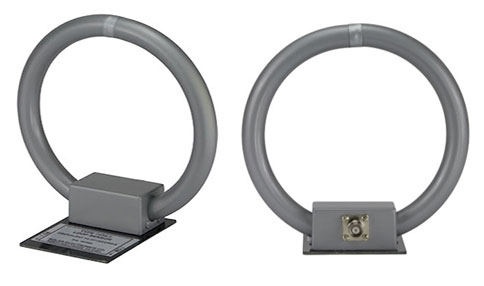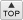# Calibration of Loop AntennasDESCRIPTIONINTRODUCTION
There are at least four different methods of calibrating shielded loop antennas. The method described here is the spaced loop technique where a loop is used as a transmitting antenna spaced a known distance from the loop being calibrated. The particular instructions given below apply specifically to the calibration of the Solar Type 7334-1 Loop Sensor used for RE01, RE101 and RE04 magnetic field emission tests (MIL-STD-461).

BASIC CONCEPT
The test setup is shown in Figure 1. The transmitting loop in this case is the Solar Type 7429-1 Loop Antenna, normally used in making RS01 magnetic field susceptibility tests.

A specific RF current is established in the transmitting loop, measured with a series resistor and a voltmeter. At a given distance away, the field intensity generated by the current in the loop can be calculated. In the setup shown, for a distance of 13.25" (33.65 cm) from the periphery of the transmitting loop winding to the center of the receiving loop winding, the field intensity is calculated to be 178 volts-per-meter when a current of 1 A is flowing in the transmitting loop.

SETUP DETAILS
Since dimensions are critical, it is important to recognize that the 13.25" (33.65 cm) is not the distance between the edges of the loops, but is from the periphery of the Type 7429-1 loop winding to the center axis of the Type 7334-1 loop. These end points are not readily determined because the Type 7429-1 loop winding is inside a piece of plastic and the center of the Type 7334-1 loop winding is difficult to determine due to the metal shield. Figure 2 shows a dimension of 10.65" (27 cm) between the faces of the loops when the 13.25" (33.65 cm) dimension is established at the theoretical points.

In setting up this dimension, take care to maintain the loops on the same axis and to orient them to face each other in a parallel fashion. A nonmetallic fixture should be used to maintain the proper physical conditions. The setup should be arranged so that no metallic objects are in the vicinity of the loop antennas. The signal generator and the EMI receiver should be at opposite ends of the setup so that the EMI receiver is not receiving the signal by some other path than by way of the Type 7334-1 loop.

ELECTRICAL DETAILS
It is not possible to obtain 1 A of RF current in the transmitting loop over the range of 30 Hz to 30 kHz with an ordinary signal generator. Fig. 1 shows the Solar Model 8850-2 Power Sweep Generator being used to deliver the required current level.

When the physical dimension is maintained and the current through the loop is adjusted at each test frequency, the voltage received by the Type 7334-1 loop is measured with the EMI receiver. In most test locations there are strong magnetic fields at the power frequency and its harmonics. It is desirable to avoid these frequencies by using a very narrow bandwidth in the EMI receiver. A simple check on the validity of the received level would be to disconnect the signal generator from the transmitting loop. If the received signal is still present, the signal is arriving at the Type 7334-1 loop from some other source.

DETERMINING LOOP FACTORS
It is desirable to obtain the correction factor for the loop in dB, since the limits of RE01 and RE101 are in terms of dB above a pT. For this reason it is convenient to measure the received voltage in dB above (or below) 1 mV.

The field intensity in this setup is 177.83 volts-per-meter (165dB/µV/meter) which equates to 115.5 dB/pT. The measured level at each frequency in dB/µV is subtracted from 165 to obtain the correction factor for field intensity in dB with reference to one microvolt-per-meter (dB/µV/m). The measured level is subtracted from 115.5 to obtain the correction factor for field intensity in dB with reference to 1 pT (dB/pT).

As an example, assume that the EMI receiver reading is 82 dB/µV at 10 kHz. Subtracting 82 from the 165 reference yields 83, the factor for field intensity in dB/ µV/m.Field Intensity:
83.0
dB/µV/m
Reference

165.0

82.0

When the answer is to be in dB/pT, derive the factor by subtracting the 82 dB in this example from 115.5 to yield a correction factor (in dB) of 33.5.

Field Intensity:
33.5
dB/pT
Reference

115.5

82.0

When using the Type 7334-1 loop in the RE01 or RE101 test, add the 33.5 correction to the EMI receiver reading (in dB) to obtain the field intensity expressed in dB/pT.

 CONVERSION TO OTHER TERMS To convert µV/m to pT, multiply by 3.33 x 10-3. To convert pT to µV/m, multiply by 3 x 102. To convert dB/µV/m to dB/pT, subtract 49.5 dB. To convert dB/pT to dB/µV/m, add 49.5 dB.

REFERENCE INFORMATION
The following expressions may be used in checking the accuracy of this test method and may be used in obtaining values for testing loop antennas of other types.

E = (47.15 N d2 l) ÷ D3

 Where: E = electric field intensity in volts-per-meter at the center of the receiving loop N = number of turns in the transmitting loop d = diameter of transmitting loop, in meters l = RF current flowing in the transmitting loop, in amperes D = hypotenuse distance "h" from the periphery of the Type 7429-1 loop winding to the center of the Type 7334-1 loop at the axis in meters